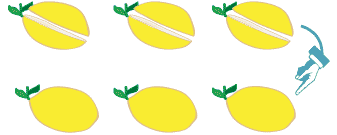## Content description

Multiply decimals by whole numbers and perform divisions by non-zero whole numbers where the results are terminating decimals, with and without digital technologies (ACMNA129)

Source: Australian Curriculum, Assessment and Reporting Authority (ACARA)

### Multiply and divide decimals

A strong understanding of the multiplication and division algorithm used with whole numbers and a clear knowledge of place value are necessary when multiplying and dividing decimal numbers.

#### Multiply decimals by whole numbers

With the introduction of decimals into the multiplication process, students need lots of experience in using materials to demonstrate what is happening.

For a multiplication such as 0.5 × 6, modelling the situation with objects, such as lemons, can help with understanding.

From working with fractions, we know that 0.5 is the same as one half. We can use lemons to show six lots of one half:Detailed description

Six lots of $$\dfrac{1}{2}$$ a lemon = three whole lemons.
6 × $$\dfrac{1}{2}$$ = 3
Six lots of 0.5 a lemon = three whole lemons.
6 × 0.5 = 3
This demonstrates that even though we are multiplying by 6, the result is smaller than 6.

For the multiplication 0.5 × 6 we might convert to fractions:

\begin{align*} 0.5 \times 6 &= \frac{1}{2} \times 6 \\ &= \frac{1}{2} \times \frac{6}{1} \\ &= \frac{6}{2} \\ &= 3 \end{align*}

\begin{align*} 0.5 \times 6 &= 0.5 + 0.5 + 0.5 + 0.5 + 0.5 + 0.5 \\ &= 3 \end{align*}

We can do a simple multiplication:

0.5 × 6 = 3.0

In our head we think 5 × 6 = 30, but the solution must have the same number of decimal places as the numbers we are multiplying. Thinking of 3.0 instead of 30 helps with this.

Or the equation could be set out using the multiplication algorithm we use for whole numbers:

 0 . 5 × 6 3 . 0

Note that there is a total of one decimal place in the two numbers being multiplied and one decimal place in the solution.

Let’s look at another example: If we buy four 2.75 litre bottles of juice, how much juice is there?

0.75 of a litre is the same as $$\dfrac{3}{4}$$ of a litre. So if we convert to fractions:

\begin{align*}2.75 \times 4 &= 2 \frac{3}{4} \times 4 \\ &= \frac{11}{4}  \times \frac{4}{1} \\ &= 11\end{align*}

 $$^3 2$$ . $$^2 7$$ 5 × 4 1 1 . 0 0